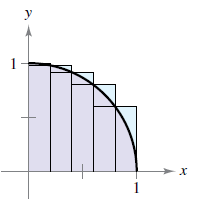Chapter 4.2, Problem 40E

Chapter
Section
Textbook Problem

Finding Upper and Lower Sums for a Region In Exercises 37-40, use upper and lower sums to approximate the area of the region using the given number of subintervals (of equal width). y = 1 − x 2To determine

To calculate: Approximate area of the provided region using upper and lower sums.

Explanation

Given: y(x)=1x2.

Formula used: Using sigma notation, the sum of n terms a1,a2,a3,,an is written as:

i=1nai=a1+a2+a3++an

Formula to calculate the lower sum:s=i=1nf(mi)Δx

where, mi are the left endpoints.

Formula to calculate upper sum:

S=i=1nf(Mi)Δx,

where, Mi are the right endpoints.

Calculation: The endpoints of the intervals and several inscribed and circumscribed rectangles are shown in the below figure.

Partition the interval [0,1] into 5 subintervals, each of width:

Δx=105=15

Left endpoints (mi),

mi=0+(i1)(15)=(i15)

where i=1,2,3,4,5.

Formula to calculate the lower sum (s):s=i=1nf(mi)Δx

where, mi are the left endpoints.

Lower sum by the left endpoints is:

s=i=15y(i15)(15)

Use value of y(i15),

s=i=15(1(i15)2)(15)

Using the formula:

i=1nai=a1+a2++an

s=[(1(115)2)(15)+(1(215)2)(15)+(1(315)2)(15)+(1(415)2)(15)+(1

Still sussing out bartleby?

Check out a sample textbook solution.

See a sample solution

The Solution to Your Study Problems

Bartleby provides explanations to thousands of textbook problems written by our experts, many with advanced degrees!

Get Started

In Exercises 1-4, simplify the expression. x2+6x+8x26x16

Calculus: An Applied Approach (MindTap Course List)

Evaluate the expression sin Exercises 116. 52535252

Finite Mathematics and Applied Calculus (MindTap Course List)

In Exercises 69-74, rationalize the numerator. 74. x2y32x

Applied Calculus for the Managerial, Life, and Social Sciences: A Brief Approach

In problems 15-26, evaluate each expression. 23.

Mathematical Applications for the Management, Life, and Social Sciences

Evaluate the integral. 28. x2+1(x22x+2)2dx

Single Variable Calculus: Early Transcendentals

Area Find the Area enclosed by the ellipse x2a2+y2b2=1. Shown in the figure.

Calculus: Early Transcendental Functions (MindTap Course List)